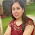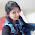## Sunday, April 06, 2014

### Prime Numbers - Properties and how to find whether a number is prime - Video Lecture

In this video I have covered all basic concepts of Prime Numbers. We all know that prime numbers are all those natural numbers that are divisible by 1 and itself and no other number.

Some properties of Prime Numbers are -

1. The smallest and the only even prime number is 2.
2. All prime numbers greater than equal to 5 are of the form 6n+1 or 6n-1. All such prime numbers that are greater than or equal to 5 leave a remainder of either 1 or 5 when divided by 6. This remainder of 5 can also be expressed in the form of 6n-1.

Also how to calculate whether a number is a prime or not is also told in this video lecture and the basic reasoning and concept why that particular method of determining whether a number is prime or not is used is partly explained in the above video tutorial and partly in the next video tutorial.

Do remember to leave your feedback through comments and you can also leave your doubts in the comment section below.

1.2.3.